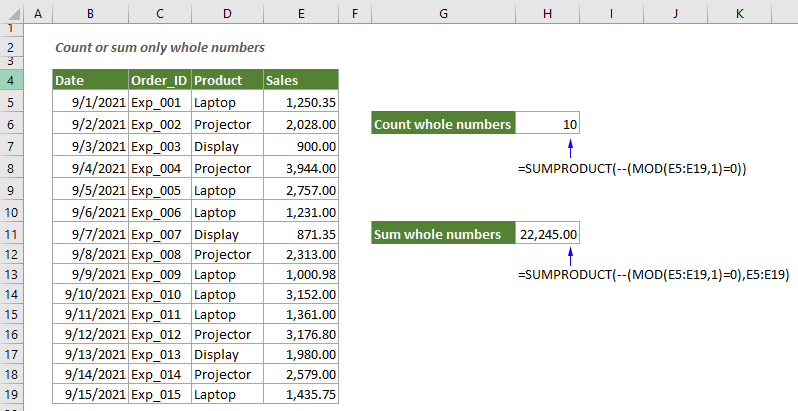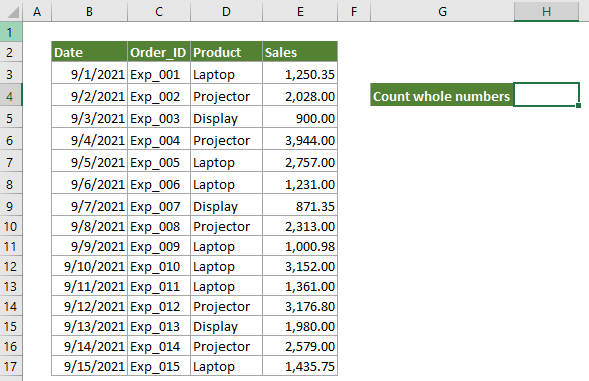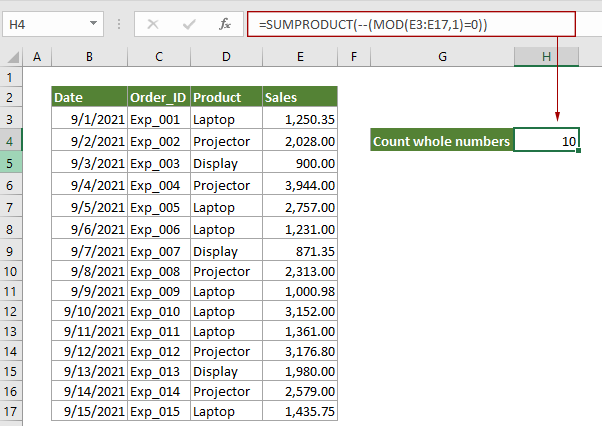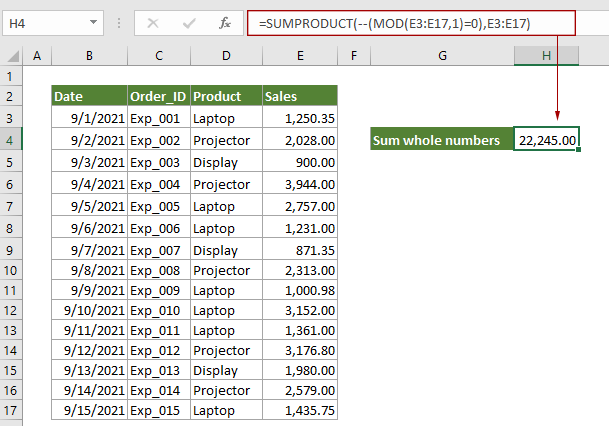## Count or sum only whole numbers in Excel

This post provides two formulas based on the SUMPRODUCT function and the MOD function to help you count and sum only whole numbers in a range of cells in Excel.How to count only whole numbers in Excel
How to sum only whole numbers in Excel

#### How to count only whole numbers in Excel

As the below screenshot shown, there is a product sales table, to count only the whole numbers in the Sales column, you can do as follow to achieve it.Generic Formula

=SUMPRODUCT(--(MOD(range,1)=0))

Arguments

Range: The range of cells you want to count whole numbers.

How to use this formula?

1. Select a blank cell to place the result.

2. Enter the below formula into it and press the Enter key to get the result.

=SUMPRODUCT(--(MOD(E3:E17,1)=0))How this formula works?

=SUMPRODUCT(--(MOD(E3:E17,1)=0))

• 1) MOD(E3:E17,1): Here use the MOD function to test for whole numbers in E3:E17. It returns the remainders after each number in range E3:E17 is divided by 1: {0.35;0;0;0;0;0.35;0;0.98;0;0;0.80;0;0;0.75}.
• 2) {0.35;0;0;0;0;0;0.35;0;0.98;0;0;0.80;0;0;0.75}=0: Compare each number in the array with 0, and returns a TRUE FALSE array like this: {FALSE;TRUE;TRUE;TRUE;TRUE;TRUE;FALSE;TRUE;FALSE;TRUE;TRUE;FALSE;TRUE;TRUE;FALSE }. In this array, TRUE values represent a whole number, and FALSE values represent a decimal number.
• 3) --{FALSE;TRUE;TRUE;TRUE;TRUE;TRUE;FALSE;TRUE;FALSE;TRUE;TRUE;FALSE;TRUE;TRUE;FALSE}: These two minus signs convert “TRUE” into 1 and “FALSE” into 0. Here you will get a new array as {0;1;1;1;1;1; 0;1;0;1;1;0;1;1;0}.
• 4) SUMPRODUCT{0;1;1;1;1;1; 0;1;0;1;1;0;1;1;0}: The SUMPRODUCT function sums all numbers in the array and returns the final result as 10.

#### How to sum only whole numbers in Excel

To sum only whole numbers in a specified range of cells, you can do as follows.

Take the above product sales table as an example.

Generic Formula

=SUMPRODUCT(--(MOD(range,1)=0)*range)

Arguments

Range: The range of cells you want to sum whole numbers.

How to use this formula?

1. Select a blank cell, enter the below formula into it and then press the Enter key to get the result.

=SUMPRODUCT(--(MOD(E3:E17,1)=0)*E3:E17)How this formula works?

=SUMPRODUCT(--(MOD(E3:E17,1)=0)*E3:E17)

• 1) MOD(E3:E17,1): Here use the MOD function to test for whole numbers in E3:E17. It returns the remainders after each number in range E3:E17 is divided by 1: {0.35;0;0;0;0;0.35;0;0.98;0;0;0.80;0;0;0.75}.
• 2) {0.35;0;0;0;0;0;0.35;0;0.98;0;0;0.80;0;0;0.75}=0: Compare each number in the array with 0, and returns a TRUE FALSE array like this: {FALSE;TRUE;TRUE;TRUE;TRUE;TRUE;FALSE;TRUE;FALSE;TRUE;TRUE;FALSE;TRUE;TRUE;FALSE }. In this array, TRUE values represent a whole number, and FALSE values represent a decimal number.
• 3) --{FALSE;TRUE;TRUE;TRUE;TRUE;TRUE;FALSE;TRUE;FALSE;TRUE;TRUE;FALSE;TRUE;TRUE;FALSE}: These two minus signs convert “TRUE” into 1 and “FALSE” into 0. Here you will get a new array as {0;1;1;1;1;1; 0;1;0;1;1;0;1;1;0}.
• 4) SUMPRODUCT({0;1;1;1;1;1;0;1;0;1;1;0;1;1;0}*E3:E17): Here each number in the array multiplies numbers in range E3:E17 to get the result as this: SUMPRODUCT(0;2028;900;3944;2757;1231;0;2313;0;3152;1361;0;1980;2579;0), and then the SUMPRODUCT function sums all numbers in the array and returns the final result as 22245.

#### Related functions

Excel SUMPRODUCT function
The Excel SUMPRODUCT function can be used to multiply two or more columns or arrays together, and then get the sum of products.

Excel MOD function
The Excel MOD function returns the remainder after number is divided by divisor. The result has the same sign as divisor.

#### Related formulas

Count numbers that begin with a specific number
This tutorial provides a formula based on the SUMPRODUCT and LEFT functions to count number of cells containing numbers that begin with a specific number(s) in Excel.

Count multiple criteria with NOT logic in Excel

Count occurrences of particular text in entire Excel workbook

Count numbers where the nth digit equals to a given number
This tutorial provides a formula based on the SUMPRODUCT and the MID function to count numbers where the nth digit equals to a given number in Excel.

### The Best Office Productivity Tools

#### Kutools for Excel - Helps You To Stand Out From Crowd

 Popular Features: Find, Highlight or Identify Duplicates  |  Delete Blank Rows  |  Combine Columns or Cells without Losing Data  |  Round without Formula ... Super VLookup: Multiple Criteria  |  Multiple Value  |  Across Multi-Sheets  |  Fuzzy Lookup... Adv. Drop-down List: Easy Drop Down List  |  Dependent Drop Down List  |  Multi-select Drop Down List... Column Manager: Add a Specific Number of Columns  |  Move Columns  |  Toggle Visibility Status of Hidden Columns  |  Compare Columns to Select Same & Different Cells ... Featured Features: Grid Focus  |  Design View  |  Big Formula Bar  |  Workbook & Sheet Manager | Resource Library (Auto Text)  |  Date Picker  |  Combine Worksheets  |  Encrypt/Decrypt Cells  |  Send Emails by List  |  Super Filter  |  Special Filter (filter bold/italic/strikethrough...) ... Top 15 Toolsets:  12 Text Tools (Add Text, Remove Characters ...)  |  50+ Chart Types (Gantt Chart ...)  |  40+ Practical Formulas (Calculate age based on birthday ...)  |  19 Insertion Tools (Insert QR Code, Insert Picture from Path ...)  |  12 Conversion Tools (Numbers to Words, Currency Conversion ...)  |  7 Merge & Split Tools (Advanced Combine Rows, Split Excel Cells ...)  |  ... and more

Kutools for Excel Boasts Over 300 Features, Ensuring That What You Need is Just A Click Away...#### Office Tab - Enable Tabbed Reading and Editing in Microsoft Office (include Excel)

• One second to switch between dozens of open documents!
• Reduce hundreds of mouse clicks for you every day, say goodbye to mouse hand.
• Increases your productivity by 50% when viewing and editing multiple documents.
• Brings Efficient Tabs to Office (include Excel), Just Like Chrome, Edge and Firefox.